#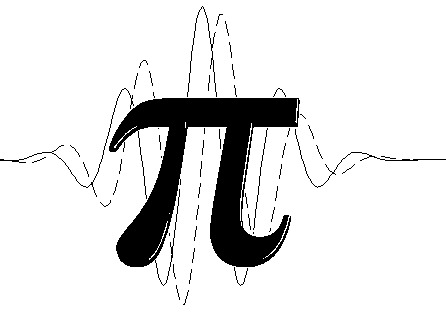PIWavelet

Python Interface for Wavelet Analysis

# PIWavelet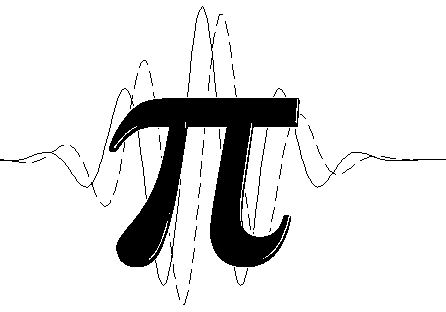Python programming Interface for Wavelet Analysis

DISCLAIMER
This module is a Python programming interface for the the matlab package for wavelet, cross-wavelet and coherence-wavelet analysis provided by Aslak Grinsted, John C. Moore and Svetlana Jevrejeva.
http://noc.ac.uk/using-science/crosswavelet-wavelet-coherence
This software may be used, copied, or redistributed as long as it is not sold and this copyright notice is reproduced on each copy made. This routine is provided as is without any express or implied warranties whatsoever.

Part of the code are originally from the project of kPyWavelet

TO RUN:

It is necessary to have gnuoctave installed in your machine Gnu Octave: http://www.gnu.org/software/octave/ (sudo apt-get install octave)

### INSTALL:

							
$python setup.py install   ### OBSERVATION: Octave supports most but not all of the core syntax and commands of MatLab. The main noticable differences are nested functions are not allowed, and GUIs (including uigetfile, etc.) are not supported. ### AUTHORS Eduardo S. Pereira. Email: pereira.somoza@gmail.com Regla D. Somoza. Email: duthit@gmail.com # Usage ### Initialization Initialization of the piwavelet class  >> import numpy as np >> from piwavelet import piwavelet   ### Wavelet Coherence Analysis: Given tow signal, with zero mean, to start the wavelet coherence analysis, it is necessary to call the wcoherence (Wavelet Coherence) class:  >> mycoherence = piwavelet.wcoherence(x,y) >> Rsq,period,scale,coi,sig95=mycoherence()   The returned values are:  Rsq Coherence Wavelet period a vector of "Fourier" periods associated with Wxy scale a vector of wavelet scales associated with Wxy coi the cone of influence sig95 Significance Plot wavelet coherence of the signals x,y.  >> mycoherence = piwavelet.wcoherence(x,y) >> mycoherence.plot(t = time, title='My Title',units='year')   Parameters :  Key Mean title (string) Title of the Plot t array with time units: (string) Units of the period and time (e.g. 'days') Optional parameters  Key Default Mean gray (boolean) True for gray map False levels List with significance level that will be showed in the plot [0.1,0.2,0.3,0.4,0.5,0.6,0.7,0.8,0.9,1.0] labels List with the Label of significance level that will be apper into the color bar. If not defined, the levels list is used instead None pArrow (boolean) True for draw vector of phase angle (it has problem not recomended for large sample of data) False pSigma (boolean) True for draw the significance contour lines True nameSave (string) path plus name to save the figure, if it is define, the plot is saved but not showed None scale (boolean) True for not log2 scale of the Plot False ### Example Consider tow stochastic signals, as presented in the following image: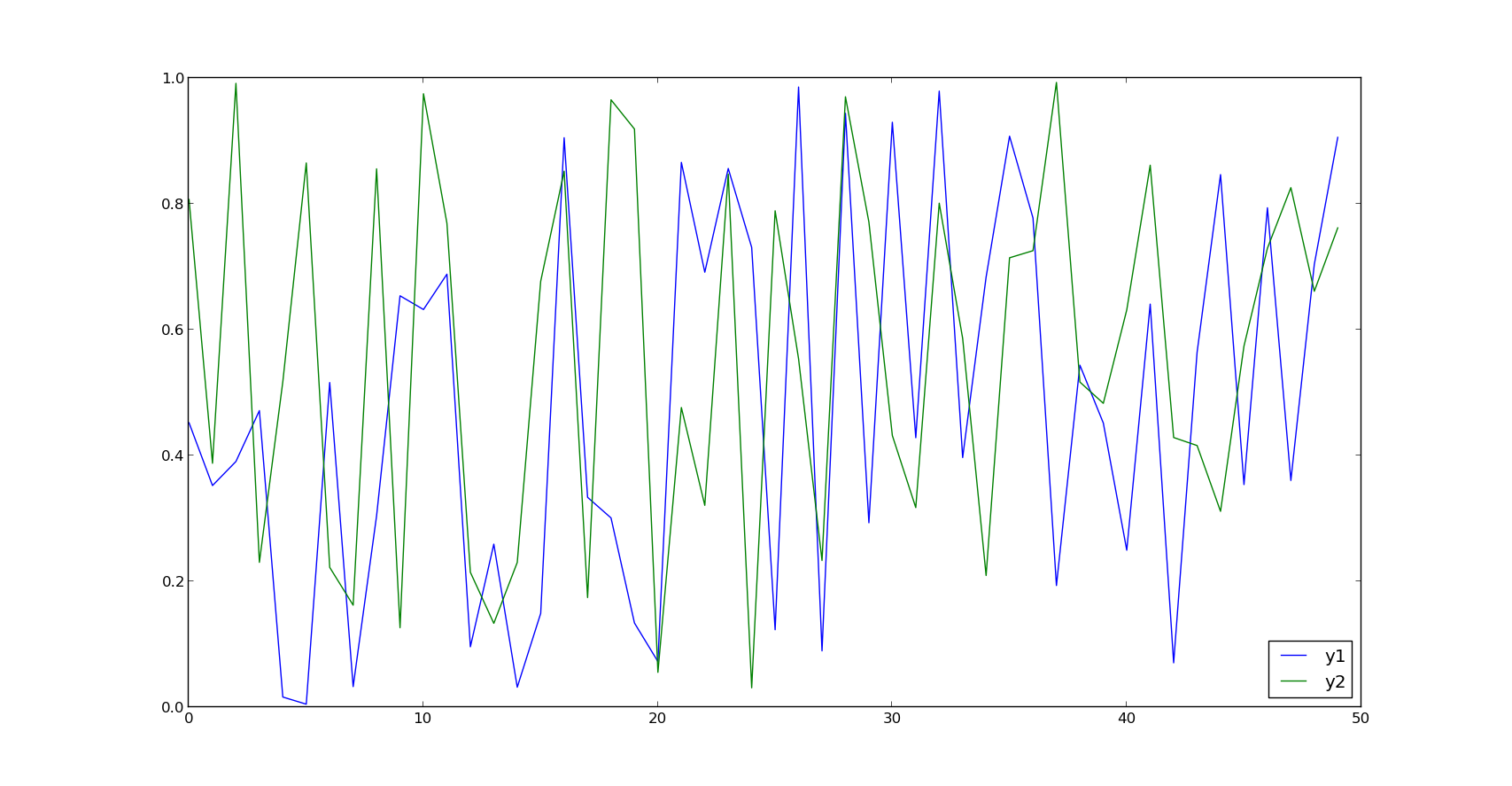The signal must be standardized with zero means. e.g. :$y_{i,std} = (y_{i}-\bar{y})/\sigma$where$y_{i}$is the$i$-th data values,$\bar{y}$is the mean value and$\sigma$is the standard deviation of the$y\$ series.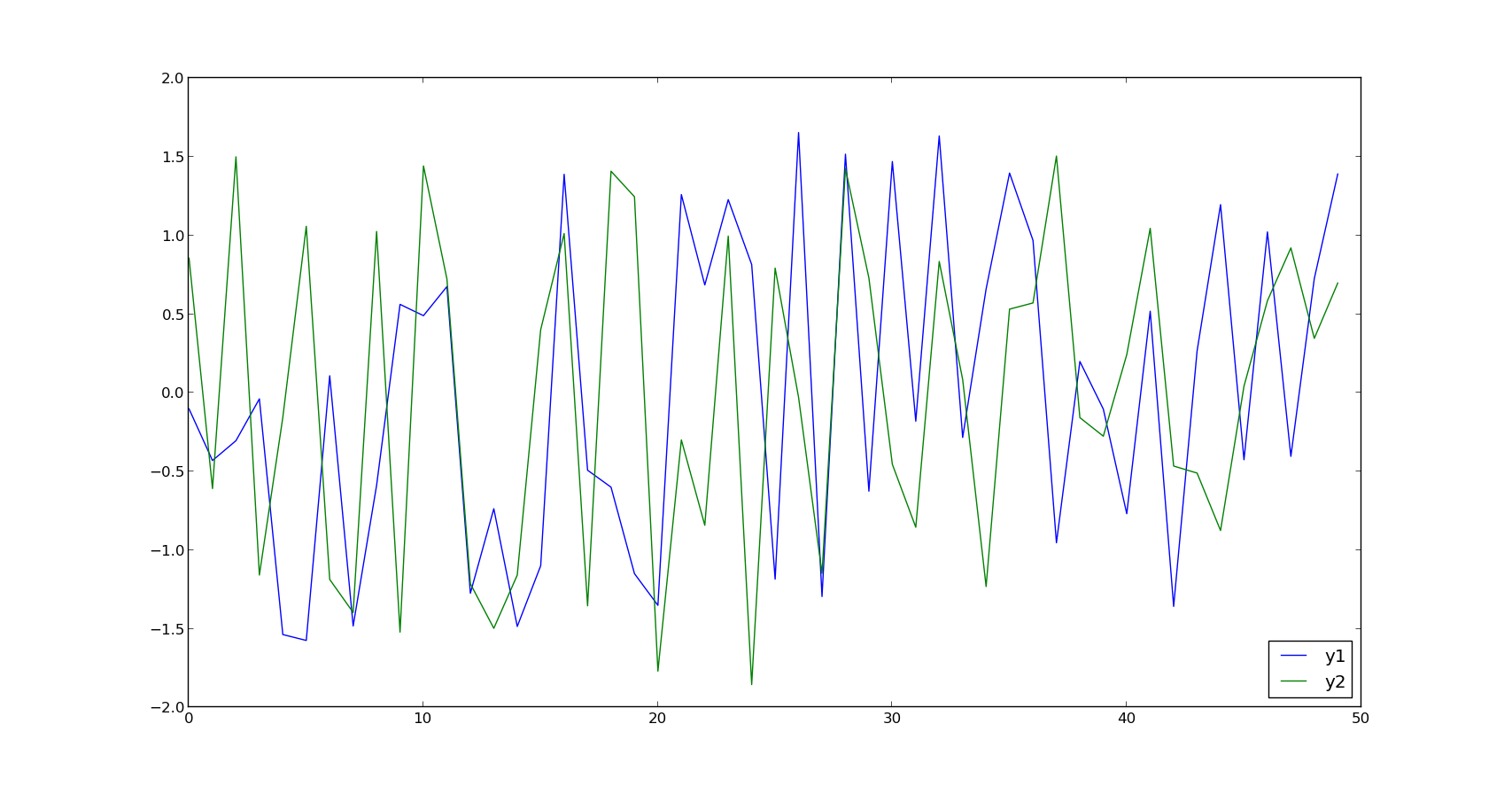The code bellow shows the coherence analysis for these signal


>>> import numpy as np
>>> from piwavelet import piwavelet
>>> y1 = np.random.rand(100) #Generation of the Random Signal 1
>>> y2 = np.random.rand(100) #Generation of the Random Signal 2
>>> x = np.arange(0,100,1) # Time step
>>> y1 = (y1-y1.mean())/y1.std() #Normalization of the Signal 1
>>> y2 = (y2-y2.mean())/y2.std() #Normalization of the Signal 2
>>> myCoherence = piwavelet.wcoherence(y1,y2) #Wavelet Coherence Analysis
>>> myCoherence.plot(t = x, title='Test',units='sec') # Plot of the Coherence Map
>>> Rsq,period,scale,coi,sig95=myCoherence() # If you want to know the individual properties.




The method plot generate the coherence map of the signal. The Figure 2 shows the result obtained from myCoherence.plot().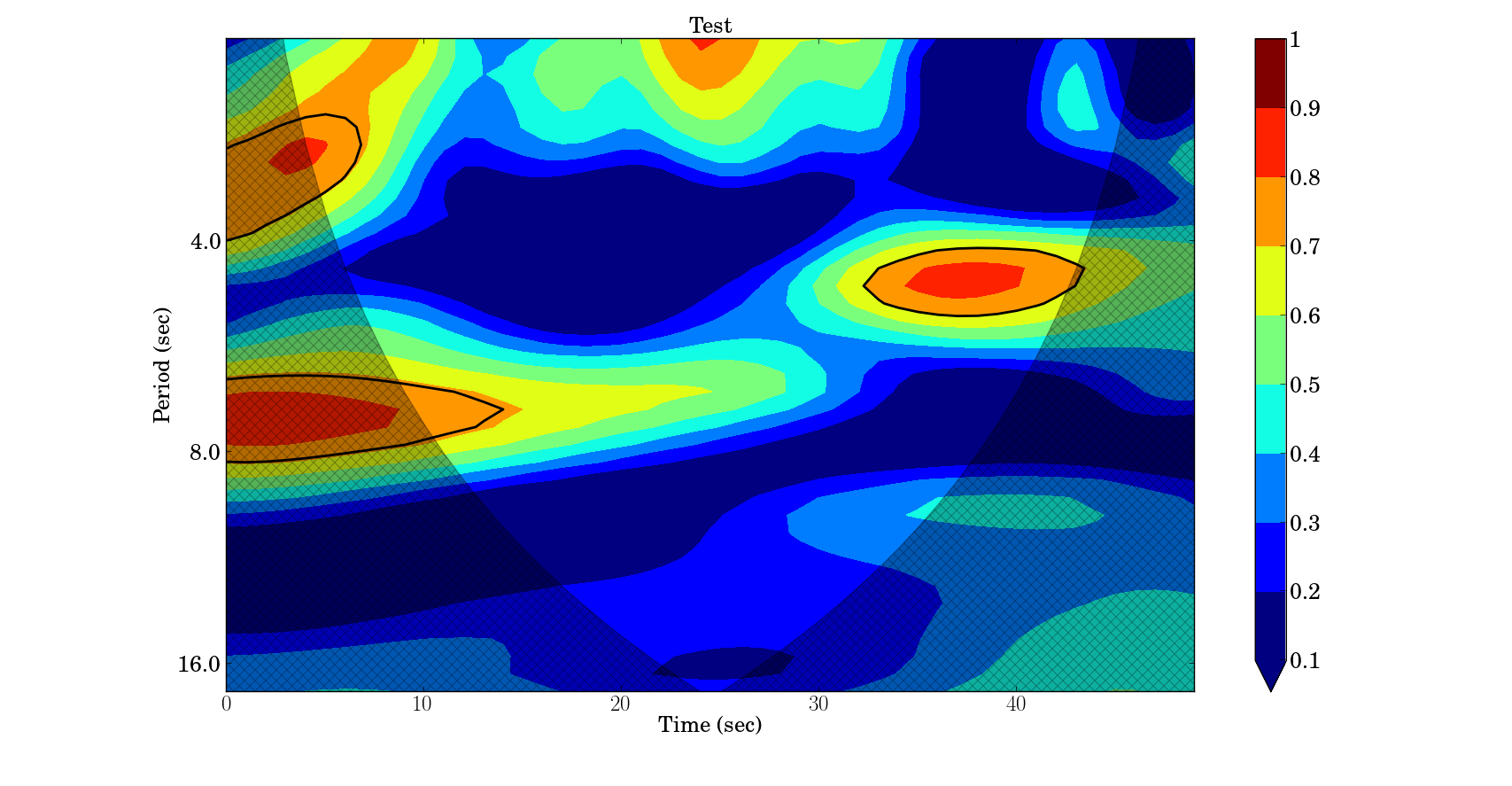### Wavelet Cross Power Spectrum Analysis:

Given tow signal, with zero mean, to start the cross wavelet analysis, it is necessary to call the wcross (Wavelet Cross Spectrum) class:


>> myXSpec = piwavelet.wcross(x,y)
>> xwt,period, scale, coi, sig95=myXSpec()



 xwt cross Wavelet period a vector of "Fourier" periods associated with Wxy scale a vector of wavelet scales associated with Wxy coi the cone of influence sig95 Significance

Plot wavelet coherence of the signals x,y.


>> myXSpec = piwavelet.wcross(x,y)
>> myXSpec.plot(t = time, title='My Title',units='year')



Parameters :

 Key Mean title (string) Title of the Plot t array with time units: (string) Units of the period and time (e.g. 'days')

Optional parameters

 Key Default Mean gray (boolean) True for gray map False levels List with significance level that will be showed in the plot [0.125, 0.25, 0.5, 1, 2, 4, 8] labels List with the Label of significance level that will be apper into the color bar. If not defined, the levels list is used instead ['1/8', '1/4', '1/2', '1', '2', '4', '8'] pArrow (boolean) True for draw vector of phase angle (it has problem not recomended for large sample of data) False pSigma (boolean) True for draw the significance contour lines True nameSave (string) path plus name to save the figure, if it is define, the plot is saved but not showed None scale (boolean) True for not log2 scale of the Plot False

#### Example

Consider the tow stochastic, standardized with zero means, signals: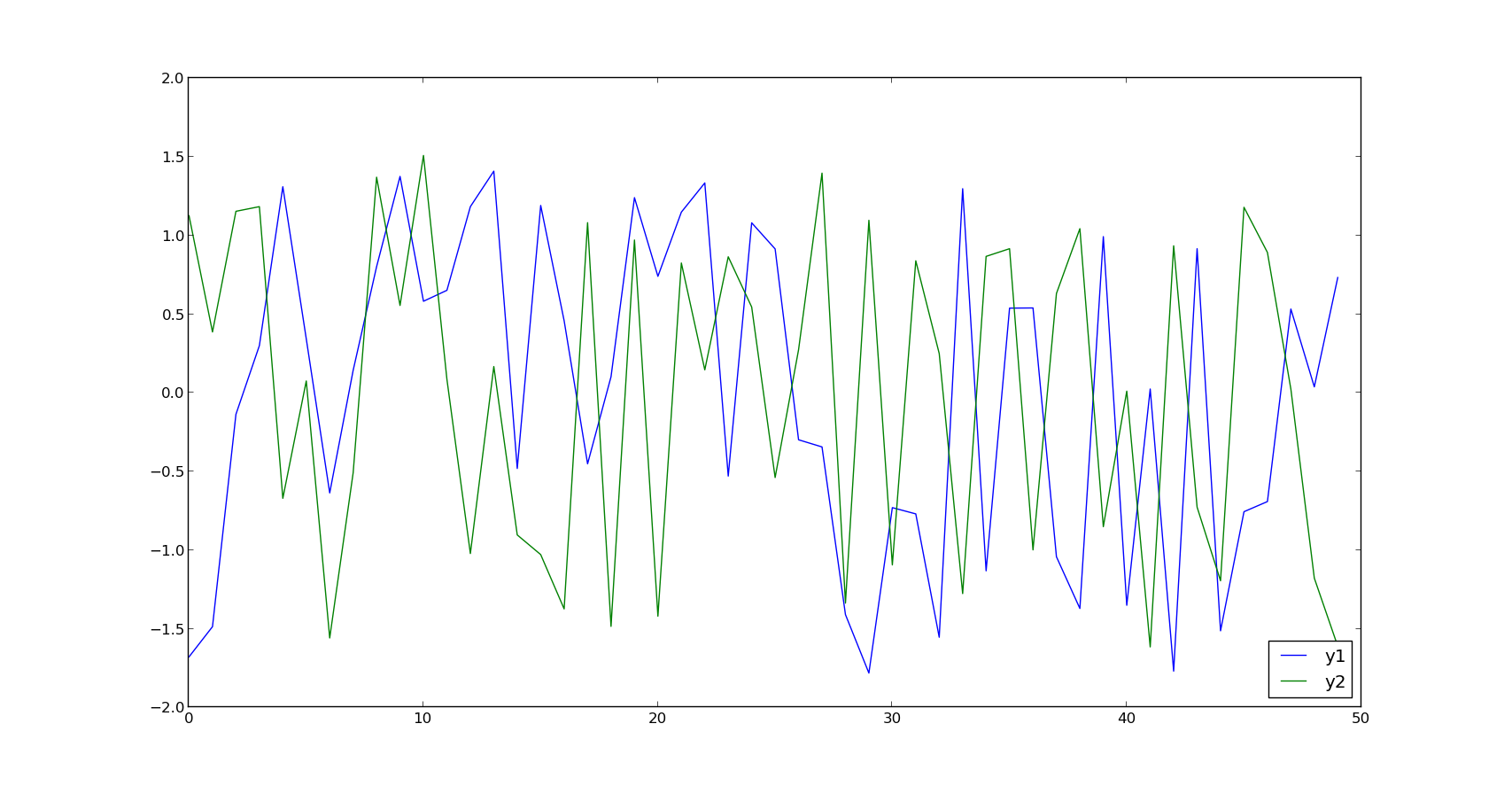The code bellow computing and plot the Cross Wavelet Power Spectrum of these signals:


>>> import numpy as np
>>> import matplotlib.pyplot as plt
>>> from piwavelet import piwavelet
>>> y1 = np.random.rand(50) #Generation of the Random Signal 1
>>> y2 = np.random.rand(50) #Generation of the Random Signal 2
>>> x = np.arange(0,50,1) # Time step
>>> y1 = (y1-y1.mean())/y1.std() #Normalization of the Signal 1
>>> y2 = (y2-y2.mean())/y2.std() #Normalization of the Signal 2
>>> myXSpec = piwavelet.wcross(y1,y2) #Cross Wavelet Analisys
>>> myXSpec.plot(t = x, title='Test',units='sec') # Plot of the Cross Power Spectrum Map
>>> xwt,period, scale, coi, sig95=myXSpec() # If you want to know the individual properties.'



The Figure 5 shows the Cross Wavelet Power Spectrum map.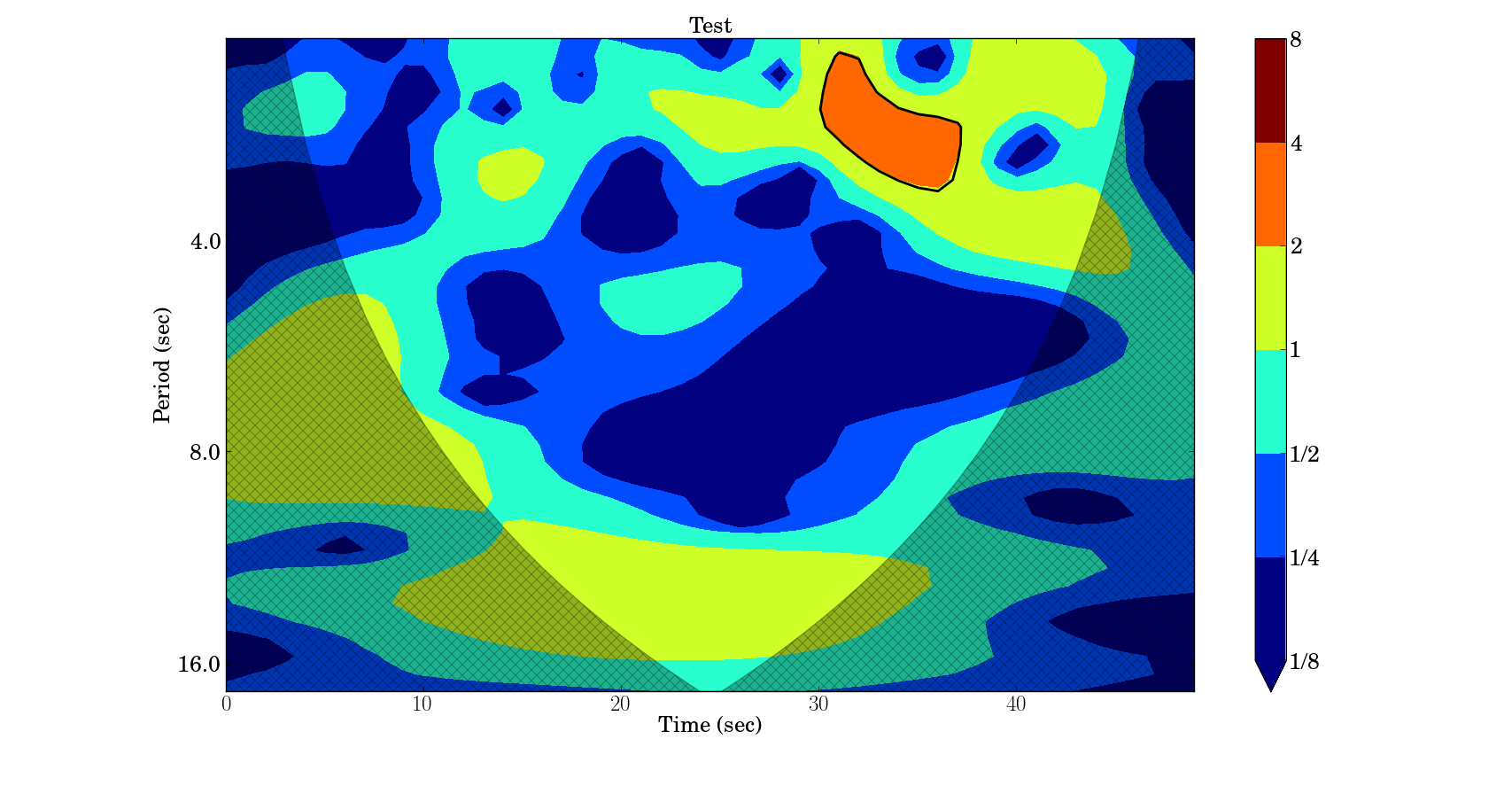### Extra Functions

#### Continues Wavelet Transform

In this package is available some wavelets mother functions (Morlet, Paul, DOG, Mexican hat), Continuous wavelet transform of the signal at specified scales, Inverse continuous wavelet transform, Significance testing for the onde dimensional wavelet transform, the Allen and Smith autoregressive lag-1 autocorrelation alpha, Lag-1 autoregressive theoretical power spectrum

These functions are originally presented in the kPyWavelet package (https://github.com/regeirk/kPyWavelet)

Also it was implemented a plotWavelet function that plot the normalized data, the wavelet transform, the power spectrum of the wavelet and the scale averaged wavelet spectrum as determined by the avg1 and avg2 parameters

Below is presented the example to run the wavelet transform (Default Morlet mother wavelet) and the plot of the wavelet.


>>> import numpy as np
>>> import matplotlib.pyplot as plt
>>> from piwavelet import piwavelet
>>> y1 = np.random.rand(50) #Generation of the Random Signal
>>> y1 = (y1-y1.mean())/y1.std() #Normalization of the Signal 1
>>> wave, scales, freqs, coi, fft, fftfreqs=piwavelet.cwt(y1) # If you want to know the individual properties.'
>>> piwavelet.plotWavelet(y1,title='test',label='Random Signal',units='days')



The Figure 6 shows the Resultant graphics.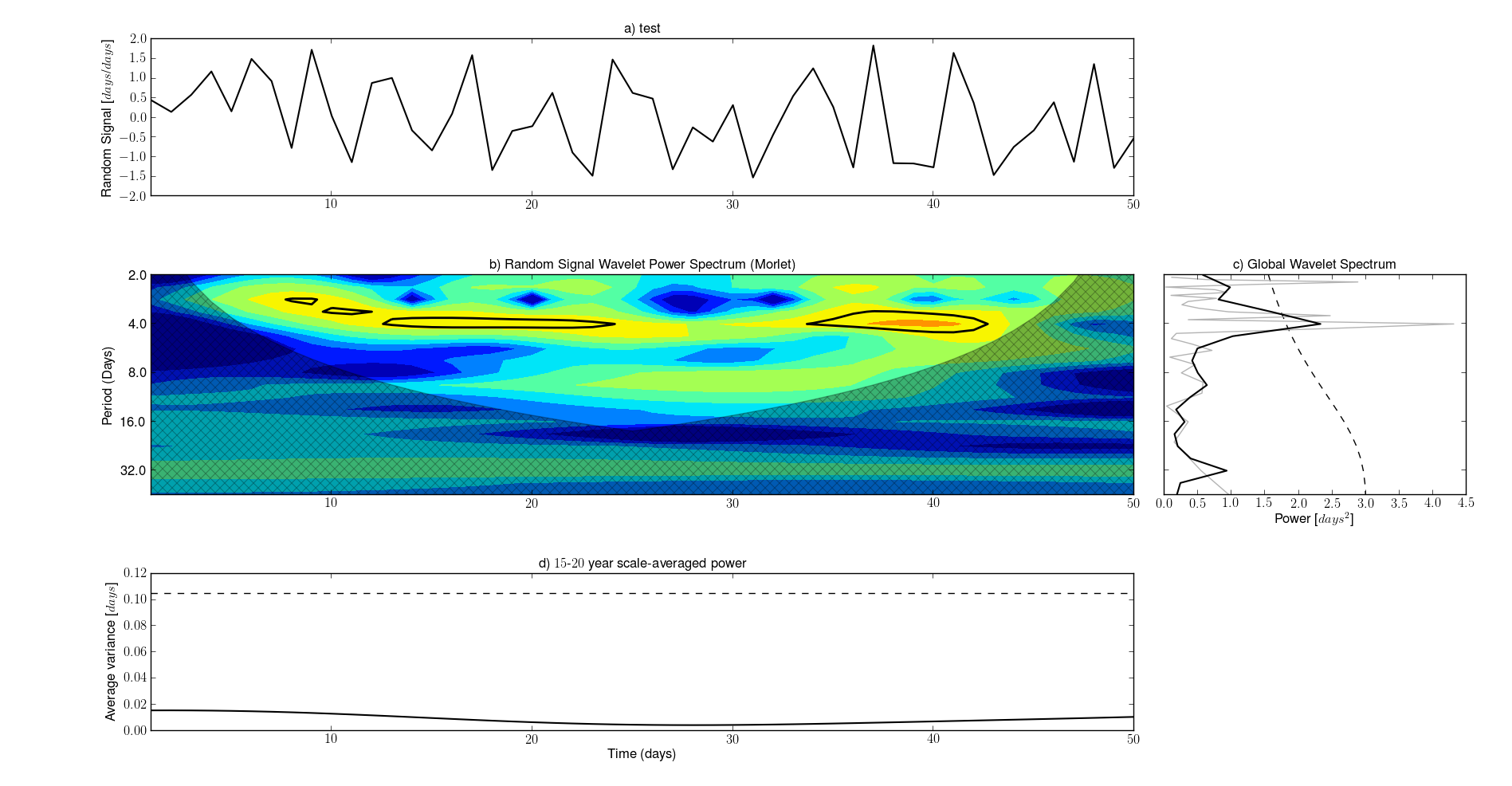### Bibliography

 Mallat, Stephane G. (1999). A wavelet tour of signal processing
 Addison, Paul S. The illustrated wavelet transform handbook
 Torrence, Christopher and Compo, Gilbert P. (1998). A Practical
Guide to Wavelet Analysis
 Grinsted, A., Moore, J.C., Jevrejeva, S., 2004,
Nonlin. Processes Geophys., 11, 561–566, doi:10.5194/npg-11-561-2004
 Jevrejeva, S., Moore, J.C., Grinsted, A., 2003,
J. Geophys. Res., 108(D21), 4677, doi:10.1029/2003JD003417
 Torrence, C., Webster, P. ,1999,
J.Clim., 12, 2679–2690

#### Credits:

Author : Eduardo S. Pereira. (pereira.somoza@gmail.com)